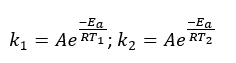# Arrhenius Equation

Chemical Kinetics: Arrhenius Equation

The Arrhenius equation is generally used to show the effect of a change of temperature on the rate constant and therefore on the rate of the reaction. If the rate constant doubles, for example, so will the rate of the reaction. An increase in temperature creates an increase in the KE of the particles. This causes more frequent collisions and increases in reaction rate. Accordingly, at a higher temperature, the fraction of collisions with enough energy equal to or greater than Ea increases. Hence, the reaction rate increases.Fig 1: The effect of temperature on the distribution of collision energy – Maxwell Boltzmann Distribution.

Recall the Maxwell Boltzmann equation.From this equation, we can see that both Ea and affect f, which in turn influences the reaction rate. However, not all collisions that occur with energy equal to, or exceeding, the activation energy lead to products. Therefore, molecular orientation is critical for reaction to occur (collision theory).

A is the frequency factor which represents the probability that collisions would occur with the proper orientation for a reaction.Here, p = orientation probability factor and Z = collision frequency. The term p is specific for each reaction and is related to the structural complexity of the reactants.

With the help of Maxwell Boltzmann Equation, Svante Arrhenius developed his theory by comparing kinetic observations with the known properties of equilibrium constants

The mathematical relationship between k and Eacan be written as:where Eais the activation energy (J/mol). R is the gas constant, R= 8.314 J/K•molor 8.314 x 10-3 kJ mol-1 K-1

And, T is the absolute temperature. The negative exponent confirms that, as T increases, the negative exponent becomes smaller (less negative), the value of k increases, and thus the reaction rate increases.

Taking the natural logarithm of both sides of the Arrhenius equation, it becomesWhen k is experimentally measured at several temperatures, Ea can be deduced from the slope of a plot of ln k vs. 1/T.Fig 2: Plot of the Arrhenius Equation.

The frequency factor, A is sometimes called the pre-exponential factor of the steric factor. It is a term which includes factors like the frequency of collisions and their orientation. It varies slightly with temperature, although not much. A is usually taken as a constant value across small temperature ranges.

Assume that for the same reaction, we have two reaction rates that are measured at a separate temperature. If data is available at two different temperatures:Write the above equation twice, once for each of the two temperatures and then subtract the lower temperature conditions from the higher temperature. The equation then becomes:Consider the following example to find Ea:

The decomposition of hydrogen iodide,

2HI(g) → H2(g) + I2(g), has rate constants of 9.51×10-9 L/mol·s at 500. K and 1.10×10-5 L/mol·s at 600. K.

We are given two rate constants and two temperatures, so we can use the Arrhenius equation to solve for Ea.= 1.76×105 J/mol = 1.76×102 kJ/molFig 3:Chemical kinetics summary.